Buscar
• edsonmontoro

# Process Reliability

This special Weibull application for production was created by Paul Barringer (1924 - 2016) and is extremely valuable to identify the causes of production losses, process variability and / or equipment breakage events, and contributes to the improvement of processes.

PS: You can check the article here by the blog or if you prefer to download the article by clicking here.

## Process Reliability

Author: Edson R. Montoro

It is very common for a process industry to have daily output as a high level performance indicator, which is evaluated every day, as it is an indication that things are going well or not.

It is also observed that it is very common to “fight” for production losses between the production and maintenance areas, because nobody wants to be the “guilty” for the losses and often the causes cannot be clearly identified, because the focus is in the witch hunt and the problem continues to occur, generating losses and more losses of production, causing damage, frustrations and worsening the organizational climate.

Paul Barringer, a reliability consultant, has developed an application of the Weibull distribution model, which we call the "concurrent causes Weibull", which clearly shows how much of the losses were caused by process variation and how much were caused by maintenance unplanned events. The method is endorsed by Dr. Abernethy in his book The New Weibull Handbook1, the author being one of the world authorities in Weibull Analysis. This book was originally sponsored by US Air Force and is used worldwide.

This method does not solve all the problems in the world, it still requires hard work and the traditional Problem Solving method. It helps to focus more on the main causes of production losses, leading to more assertive actions contributing to the elimination and/or minimization of production losses, lowering production costs and improving the climate within the organization.

The method consists of collecting the daily production values ​​and plotting them in a Weibull probability paper, using their own application. It is noteworthy that not all commercial applications have the Weibull function for competing causes, and those that have it usually do not have the Process Reliability function and the calculations must be done manually.

There is an application that has this function in a very structured way, which is SuperSMITH Weibull 5.0 created by Fulton Finding, presented in Figure 1.Figure 1 - Software Interface (superSmith Weibull - version 5.0).

To demonstrate the application of Process Reliability through the Weibull probability distribution model, Weibull's basic concepts will be presented to demonstrate how to interpret a graph of daily productions.

The random variable Daily Production is normally not explained by the Normal probability distribution model, because on the right we have a physical limitation of the plant's installed production capacity, that is, we have no values higher than this limit, which makes the distribution truncated. As production losses occur, we will have lower production values, making the distribution shape elongated to the left, which can be seen in Figure 2.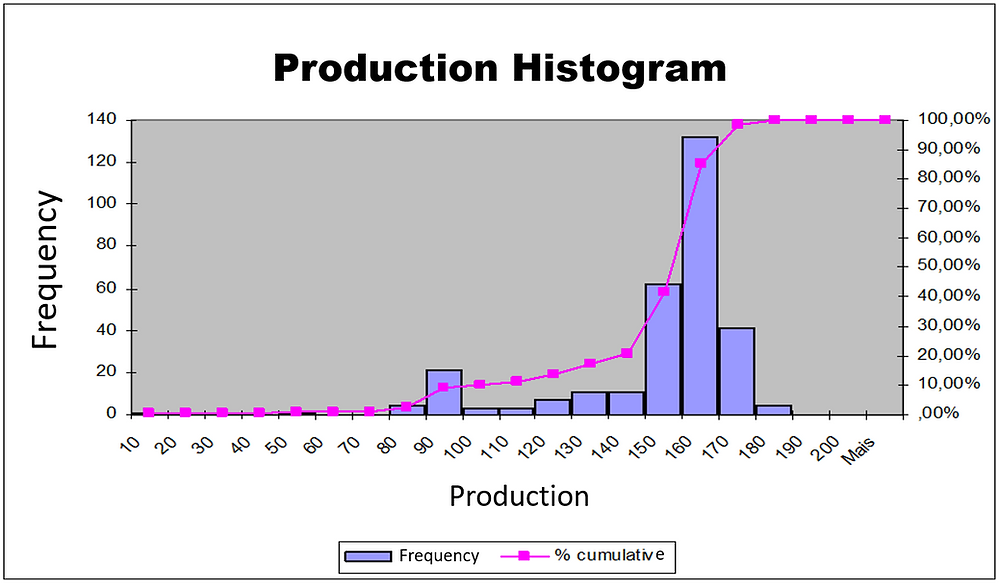Figure 2 – Daily Production Histogram.

This distribution behavior is well explained by the Weibull model, originally proposed by Waloddi Weibull , and can be described by the cumulative distribution function presented by Function 1.Where,

F (t) = cumulative distribution function;

t = variable evaluated by the function, in this case it is the daily production;

b = shape parameter - slope of the curve on the Weibull probability paper;

h = scale parameter (Characteristic Life) - 63.2% of the values are below it.

There are infinite formats of the Weibull distribution. You can see some examples in Figure 3, which presents some Probability Distribution Functions.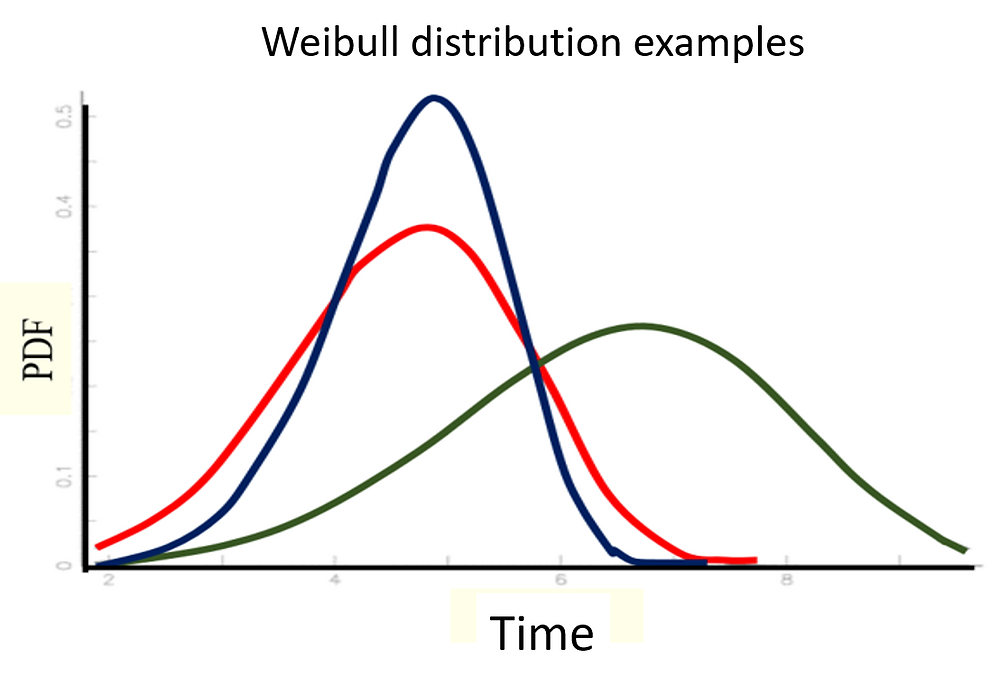Figure 3 - Some formats of Weibull distributions.

Weibull's distribution model requires many hours of study and learning, but only the concepts important to the object of study, i.e: production losses, will be presented here.

Weibull’s, Beta (b) e Eta (h)parameters are respectively called shape and scale (characteristic life) parameters, with the latter reporting that 63.2% of daily production values were below it, which leads to the conclusion that the higher this parameter, the better for production.

We can roughly compare Weibull's Beta (b) and Eta (h) parameters with the Mean and Standard Deviation in the Normal model.

The curve shape (b) also helps to understand the random variable of interest: Daily Production. For example, the higher the value, the lower the variability, and it is assumed, according to Barringer, that a value of (b) = 75 corresponds to a level 6 Sigma process.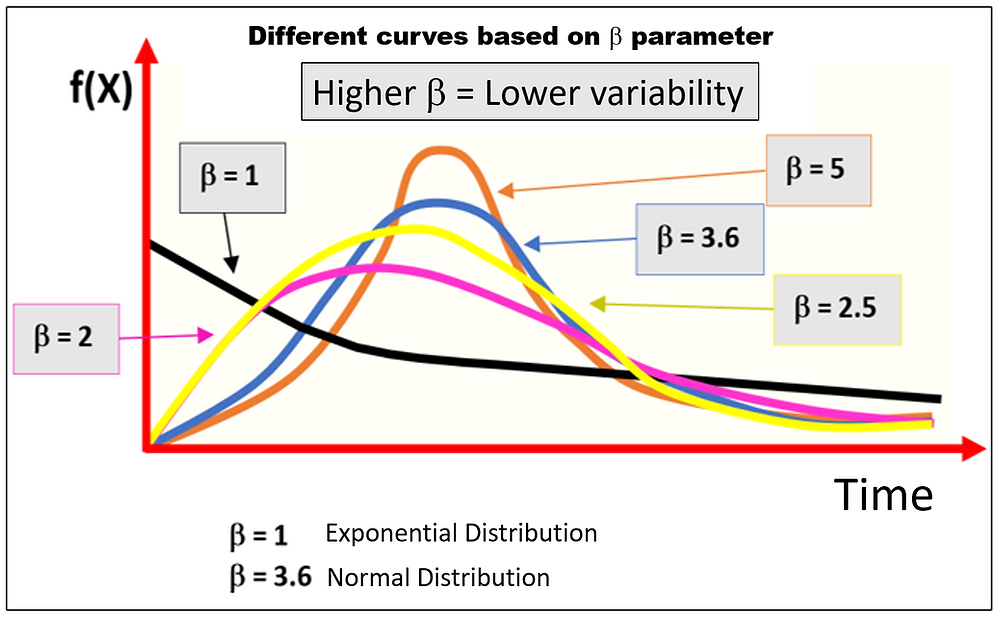Figure 4 - Curves with different shape parameters (b).

The Reliability function R(t) also depends on the shape of the curve (b), among other factors. The higher the parameter b the higher the reliability, which also reinforces the fact that the higher this parameter, the better the production. We can see this relationship in Figure 5.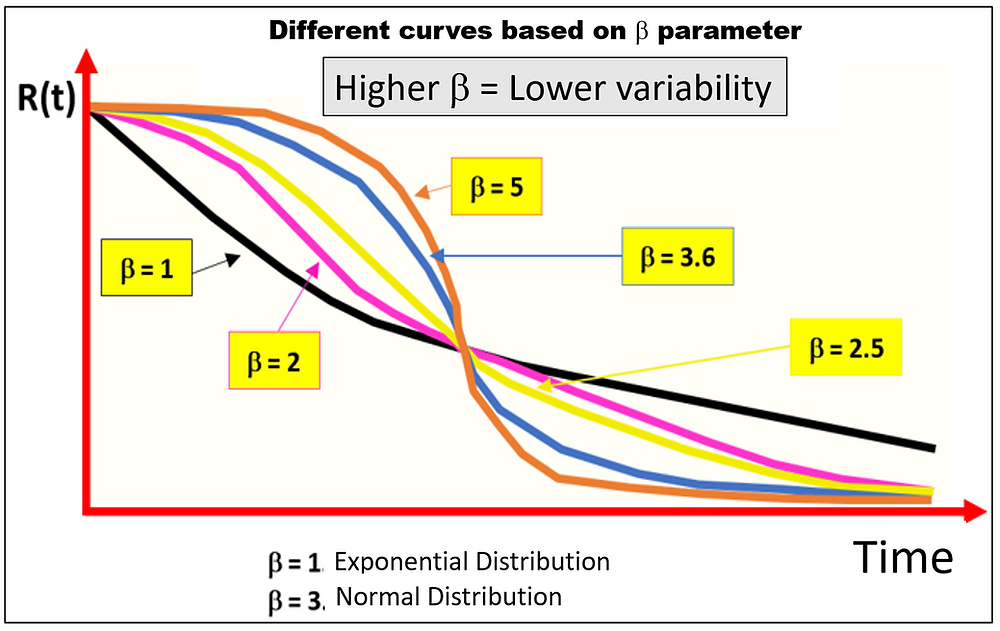Figure 5 - Relationship of (b) with reliability.

Daily Production results are plotted on a Weibull's probability paper (WPP) for analysis and loss calculations. WPP's function is to turn the Weibull curve into a straight line for ease of interpretation. This transformation is simply to linearize the Weibull function by applying logarithm twice, obtaining Function 2.This function (Function 2) has the general shape of a linear function of type Y = aX + b, a line, as shown in Figure 6, where we have different Betas (b), which correspond to different slope of lines.

It is possible to relate the two functions (Weibull logarithmized function and line function) through the following relations:Figure 6 shows different Weibull curves with different(b) inclinations.Figure 6 - Comparison among different betas.

The concept of process reliability is used with the Weibull probability paper, where the daily production logarithm on the horizontal axis is plotted against the double Weibull probability logarithm on the vertical axis, thus obtaining the Demonstrated Production curve. One more curve is constructed: the Nameplate Production or Design curve, with a slope (β) of 75, since Barringer considers that this variability corresponds to a level 6 Sigma process. The visualization of these curves can be observed in Figure 7.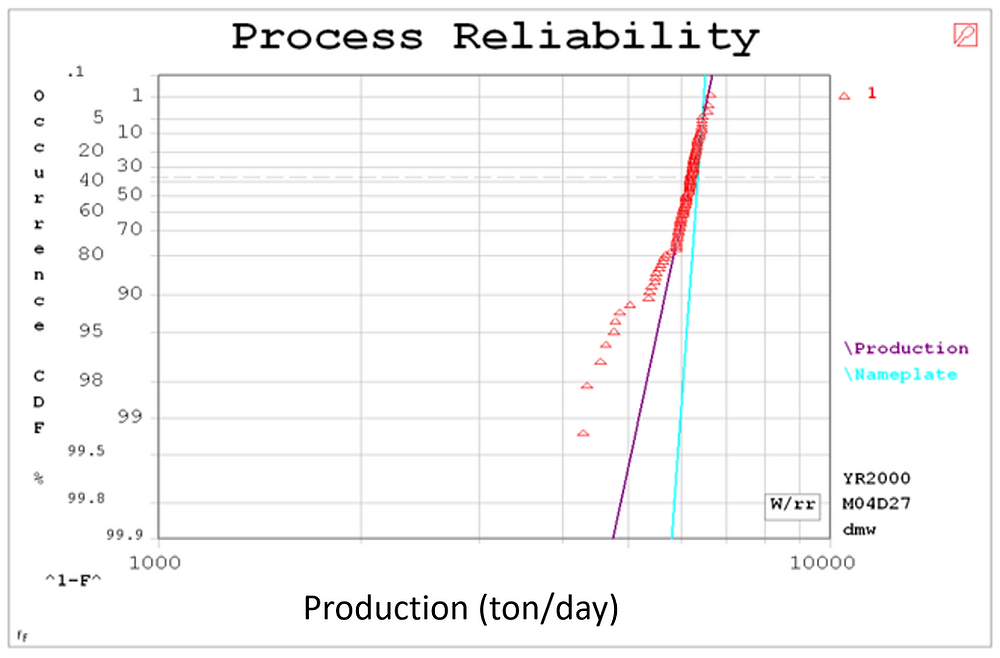Figure 7 – Weibull’s probabilities Paper showing both curves.

To extract all the information, perform the analysis, and provide improvement actions, you need to introduce the following concepts (shown in Figure 8) described below.Figure 8 – Weibull’s Probabilities Paper showing all information.

As per figure 8:

Demonstrated Production Line - These are the actual results of Daily Production. In the example in Figure 8, it has a b of 26 and a h of 6186, ie 63.2% probability of producing 6186 tons or less daily.

Nameplate Line - Consider the highest production value and draw a line with a slope (b) of 75, as noted earlier.

h Line - Represents the probability of 63.2%, that by cutting the two lines (Demonstrated Production and Nameplate lines) and projecting on the horizontal axis it is possible to obtain the values ​​of h for both curves.

Process Reliability - This is the point at which the Demonstrated Production curve abruptly changes slope. It informs you of the likelihood of a good production day without flow cuts or worse. It also represents that from this point on, production values ​​are related to special causes of variation.

Cutback losses area - This is the region in which points represent production cuts caused by a variety of situations and highlighting losses.

Production losses by equipment - This is the sum of the distances between the points and the Demonstrated Production curve (region shown in Figure 8).

Crash & Burn Points - These correspond to low production days, usually caused by equipment problems.

Efficiency & Utilization Losses - This is the sum of the differences between the Demonstrated Production and Nameplate curve points, caused primarily by process variability.

SuperSmith Weibull software (version 5.0) provides a summary of the calculated losses and the parameter values ​​of the two curves, as shown in Figure 9.Figure 9 - Example of loss and information summary (software output).

With this information, it is clearer to see if production losses are caused by process variability (linked to operation), or due to equipment problems (linked to maintenance).

In the example (Figure 9), losses caused by process variability (Efficiency + Utilization) of 7504,268 units are greater than losses caused by equipment breakdown (1045.94 units), so the focus should be on improving control of the process.

Still in this example, Process Reliability is 90.47%, indicating a high reliability, that is, it shows 90.47% chance of the process to work without equipment downtime.

Thus, the actions to eliminate problems are faster and more assertive, thus contributing to the continuous improvement of processes.

It is reinforced that the tool is not magic, and practice is required. It should not be forgotten that Problem Solving techniques help to speed up problem solving, contributing to the appreciation of the professionals involved.

## Referências:

(1) Abernethy, R. B., The New Weibull Handbook, 5th edition; Abernethy, North Palm Beach, 1996.

(2) Weibull, E. H. W., “A Statistical Theory of The Strength of Materials”, Ingeniörsvetenskapsakademiens Handlingar Nr151, Generalstabens Litografiska Anstalts Förlag, Stockholm, 1939.

Edson R. Montoro is Technical Director of ERMontoro Consulting and Training Ltda, a company focused on people development and process improvement consulting using Applied Statistics and Lean Manufacturing.

The author is a Chemist from UNESP (Paulista State University “Júlio de Mesquita Filho) - Araraquara, MBA in Business Management from FGV (Getulio Vargas Foundation), Master Black Belt from Air Academy Associates, Quality Engineer from ASQ (America Society for Quality) and Postgraduate in Production Management from UFSC (Federal University of Santa Catarina).

292 visualizações0 comentário

Ver tudo